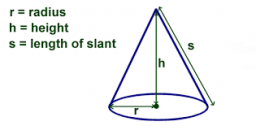# Calculate 65804

Calculate the surface and volume of a rotating cone, the base of which has a diameter of 6 cm and its height of 4 cm.

S =  75.3982 cm2
V =  37.6991 cm3

### Step-by-step explanation:Did you find an error or inaccuracy? Feel free to write us. Thank you!

Tips for related online calculators
Need help calculating sum, simplifying, or multiplying fractions? Try our fraction calculator.
Tip: Our volume units converter will help you convert volume units.

#### Grade of the word problem:

We encourage you to watch this tutorial video on this math problem: